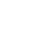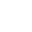> > Ellipse

# Ellipse

The set of all points in a plane, the sum of whose distances from two fixed points in the plane is constant is an ellipse. These two fixed points are the foci of the ellipse (Fig. 1). When a line segment is drawn joining the two focus points, then the mid-point of this line is the center of the ellipse.

### Suggested VideosIntroduction to Conic SectionsTerms Associated to ConicsDiameter of a ParabolaThis line joining the two foci is called the major axis and a line drawn through the center and perpendicular to the major axis is the minor axis. The endpoints of the major axis are called vertices of the ellipse (Fig. 2).Also, we denote

• The length of the major axis by ‘2a’
• Length of the minor axis by ‘2b’
• The distance between the foci by ‘2c’.### Browse more Topics under Conic Sections

Hence,

• The length of the semi-major axis is ‘a’
• And the length of the Semi-minor axis is ‘b’
• Further, the distance of focus from the center is ‘c’

##### The Relationship Between ‘a’, ‘b’, and ‘c’

Take a look at the following diagram:As shown, take a point P at one end of the major axis. Hence, the sum of the distances between the point P and the foci is,

F1P + F2P = F1O + OP + F2P = c + a + (a–c) = 2a.

Next, take a point Q at one end of the minor axis. Now, the sum of the distances between the point Q and the foci is,

F1Q + F2Q = √ (b2 + c2) + √ (b2 + c2) = 2√ (b2 + c2)

We know that both points P and Q are on the ellipse. Hence, by definition we have

2√ (b2 + c2) = 2a
Or, √ (b2 + c2) = a
i.e. a2 = b2 + c2 or c2 = a2 – b2

### Special Cases

In the equation, c2 = a2 – b2, if we keep ‘a’ constant and vary the value of ‘c’ from ‘0-to-a’, then the resulting ellipses will vary in shape.

• Case-I c = 0: When c = 0, both the foci merge at the center of the figure. Also, a2 becomes equal to b2, i.e. a = b. Hence, the ellipse becomes a circle.
• Case-II c = a: When c = a, b = 0. Hence, the ellipse reduces to a line joining the two points F1 and F2.

### Eccentricity

It is the ratio of the distances from the center of the ellipse to one of the foci and one of the vertices of the ellipse. It is denoted by ‘e’. Therefore, e = c/a.

## Standard Equations of Ellipse

When the center of the ellipse is at the origin and the foci are on the x-axis or y-axis, then the equation of the ellipse is the simplest. Here are two such possible orientations:Of these, let’s derive the equation for the ellipse shown in Fig.5 (a) with the foci on the x-axis. Let F1 and F2 be the foci and O be the mid-point of the line F1F2. Also, let O be the origin and the line from O through F2 be the positive x-axis and that through F1 as the negative x-axis.

Further, let the line drawn through O perpendicular to the x-axis be the y-axis. Let the coordinates of F1 be (– c, 0) and F2 be (c, 0) as shown in Fig.5 (a) above.

## Derivation of Equations of Ellipse

Now, we take a point P(x, y) on the ellipse such that, PF1 + PF2 = 2a

By the distance formula, we have,
√ {(x + c)2 + y2} + √ {(x – c)2 + y2} = 2a
Or, √ {(x + c)2 + y2} = 2a – √ {(x – c)2 + y2}

Further, let’s square both sides. Hence, we have
(x + c)2 + y2 = 4a2 – 4a√ {(x – c)2 + y2} + (x – c)2 + y2

Simplifying the equation, we get √ {(x – c)2 + y2} = a – x(c/a)
We square both sides again and simplify it further to get,
x2/a2 + y2/(a2 – c2) = 1

We know that c2 = a2 – b2. Therefore, we have x2/a2 + y2/b2 = 1
Therefore, we can say that any point on the ellipse satisfies the equation:

x2/a2 + y2/b2 = 1 … (1)

### Converse Situation

Let’s look at the converse situation now. If P(x, y) satisfies equation (1) with 0 < c < a, then y2 = b2(1 –( x2/a2))

Therefore, PF1 = √ {(x + c)2 + y2}
= √ {(x + c)2 + b2(1-(x2/a2))}

Simplifying the equation and replacing b2 with a2 – c2, we get PF1 = a + x(c/a)
Using similar calculations for PF2, we get PF2 = a – x(c/a)
Hence, PF1 + PF2 = {a + x(c/a)} + {a – x(c/a)} = 2a.

Therefore, any point that satisfies equation (1), i.e. x2/a2 + y2/b2 = 1, lies on the ellipse. Also, the equation of an ellipse with the centre of the origin and major axis along the x-axis is:

x2/a2 + y2/b2 = 1.

Note: Solving the equation (1), we get

x2/a2 = 1 – y2/b2 ≤ 1

Therefore, x2 ≤ a2. So, – a ≤ x ≤ a. Hence, we can say that the ellipse lies between the lines x = – a and x = a and touches these lines. Similarly, it can be between the lines y = – b and y = b and touches those lines. Its equation {Fig. 5 (b)} is:

x2/b2 + y2/a2 = 1.

Hence the Standard Equations of Ellipses are:

• x2/a2 + y2/b2 = 1.
• x2/b2 + y2/a2 = 1.

### Observations

• An ellipse is symmetric to both the coordinate axes. In simple words, if (m, n) is a point on the ellipse, then (- m, n), (m, – n) and (- m, – n) also fall on it.
• The foci always lie on the major axis.
• If the coefficient of x2 has a larger denominator, then the major axis is along the x-axis.
• If the coefficient of y2 has a larger denominator, then the major axis is along the y-axis.

## Latus Rectum of Ellipse

Latus rectum of an ellipse is a line segment perpendicular to the major axis through any of the foci and whose endpoints lie on the ellipse as shown below.Let’s find the length of the latus rectum of the ellipse x2/a2 + y2/b2 = 1 shown above. Let the length of AF2 be l. Therefore, the coordinates of A are (c, l).

∴ x2/a2 + y2/b2 = c2/a2 + l2/b2 = 1

Now, in ‘eccentricity’ we learned that e = c/a or c = ae. Substituting the values, we get

(ae)2/a2 + l2/b2 = 1
Or, e2 + (l2/b2) = 1
l2 = b2(1 – e2)

Now, we know that e = c/a
∴ e2 = c2/a2 = (a2 – b2)/a2 …(from the relationship between a, b, and c) = 1 – b2/a2

Hence, we have
l2 = b2(1 – e2) = b2(1 – {1 – b2/a2}) = b2(1 – 1 + b2/a2) = b4/a2
Or, l = b2/a

Since the ellipse is symmetric to the y-axis,

AF2 = F2B

So, the length of the latus rectum is = 2b2/a.

## Solved Examples for You

Q 1: Find the coordinates of the foci, vertices, lengths of major and minor axes and the eccentricity of the ellipse 9x2 + 4y2 = 36.

A: Given, 9x2 + 4y2 = 36. Dividing both sides by 36, we get

x2/4 + y2/9 = 1

Observe that the denominator of y2 is larger than that of x2. Hence, the major axis is along the y-axis. Now, comparing it with the standard equation, we get, a2 = 4 or a = 2 and b2 = 9 or b = 3

Also, c2 = a2 – b2
Or, c = √ (a2 – b2) = √ (9 – 4) = √5
And, e = c/a = √5/3

Therefore,

• The foci are (0, √5) and (0, – √5).
• Vertices are (0, 3) and (0, – 3)
• Length of the major axis = 6
• Length of the minor axis = 4
• Eccentricity = √5/3

Q 2: What are the types of an ellipse?

A: Basically, there are two major kinds of ellipses. One is the horizontal major axis ellipse and the other is vertical major axis ellipse.

Q 3: What is the equation of ellipse?

A: (x^2/a^2)+(y^2/b^2)=1.

Q 4: Tell about the two foci of an ellipse.

A:  The two prominent points on every ellipse are the foci. Further, there is a positive constant 2a which is greater than the distance between the foci. From any point, the sum of the distances to the two foci is equal to 2a.

Q 5: What is an ellipse shape?

A: An ellipse is a shape that resembles an oval or a flattened circle. In geometry, an ellipse is a plane curve resulting from the intersection of a cone by a plane in a way that creates a closed curve. Moreover, a circle is a special case of an ellipse.

Share with friends

## Customize your course in 30 seconds

##### Which class are you in?
5th
6th
7th
8th
9th
10th
11th
12th
Get ready for all-new Live Classes!
Now learn Live with India's best teachers. Join courses with the best schedule and enjoy fun and interactive classes.Ashhar Firdausi
IIT Roorkee
BiologyDr. Nazma Shaik
VTU
ChemistryGaurav Tiwari
APJAKTU
Physics
Get Started

## Browse

##### Conic Sections2 Followers

Most reacted comment
1 Comment authorsRecent comment authors
Subscribe
Notify ofGuest
Dami

Nigeria not among

## Question Mark?

Have a doubt at 3 am? Our experts are available 24x7. Connect with a tutor instantly and get your concepts cleared in less than 3 steps.